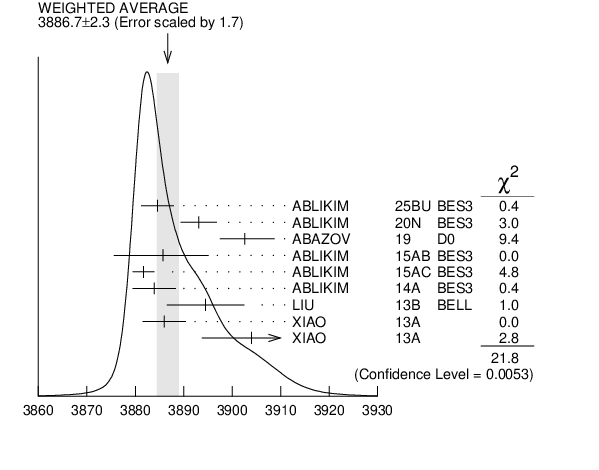#### ${{\boldsymbol Z}_{{c}}{(3900)}}$ MASS

VALUE (MeV) EVTS DOCUMENT ID TECN CHG  COMMENT
 $\bf{ 3887.1 \pm2.6}$ OUR AVERAGE  Error includes scale factor of 1.7. See the ideogram below.
$3893.1$ $\pm2.2$ $\pm3.0$ 1
 2020 N
BES3 0 ${{\mathit e}^{+}}$ ${{\mathit e}^{-}}$ $\rightarrow$ ${{\mathit \pi}^{0}}{{\mathit \pi}^{0}}{{\mathit J / \psi}}$
$3902.6$ ${}^{+5.2}_{-5.0}$ ${}^{+3.3}_{-1.4}$ 2, 3
 2019
D0 $\pm{}$ 1.96 TeV ${{\mathit p}}$ ${{\overline{\mathit p}}}$ $\rightarrow$ ${{\mathit J / \psi}}{{\mathit \pi}^{+}}{{\mathit \pi}^{-}}{{\mathit X}}$
$3881.2$ $\pm4.2$ $\pm52.7$ 6k 4
 2017 J
BES3 $\pm{}$ ${{\mathit e}^{+}}$ ${{\mathit e}^{-}}$ $\rightarrow$ ${{\mathit \pi}^{+}}{{\mathit \pi}^{-}}{{\mathit J / \psi}}$
$3885.7$ ${}^{+4.3}_{-5.7}$ $\pm8.4$ 2, 4
 2015 AB
BES3 0 ${{\mathit e}^{+}}$ ${{\mathit e}^{-}}$ $\rightarrow$ ${{\mathit \pi}^{0}}$( ${{\mathit D}}{{\overline{\mathit D}}^{*}}$) ${}^{0}$
$3881.7$ $\pm1.6$ $\pm1.6$ 1.2k 2, 4
 2015 AC
BES3 $\pm{}$ ${{\mathit e}^{+}}$ ${{\mathit e}^{-}}$ $\rightarrow$ ${{\mathit \pi}^{\pm}}$( ${{\mathit D}}{{\overline{\mathit D}}^{*}}$) ${}^{-+}$
$3883.9$ $\pm1.5$ $\pm4.2$ 1.2k 2, 4
 2014 A
BES3 $\pm{}$ ${{\mathit e}^{+}}$ ${{\mathit e}^{-}}$ $\rightarrow$ ${{\mathit \pi}^{\pm}}$ ( ${{\mathit D}}{{\overline{\mathit D}}^{*}}$ )${}^{-+}$
$3894.5$ $\pm6.6$ $\pm4.5$ 159 2
 2013 B
BELL $\pm{}$ ${{\mathit e}^{+}}$ ${{\mathit e}^{-}}$ $\rightarrow$ ${{\mathit \gamma}}{{\mathit \pi}^{+}}{{\mathit \pi}^{-}}{{\mathit J / \psi}}$
$3886$ $\pm4$ $\pm2$ 81 2, 5
 2013 A
$\pm{}$ 4.17 ${{\mathit e}^{+}}$ ${{\mathit e}^{-}}$ $\rightarrow$ ${{\mathit \pi}^{+}}{{\mathit \pi}^{-}}{{\mathit J / \psi}}$
$3904$ $\pm9$ $\pm5$ 25 2, 5
 2013 A
0 4.17 ${{\mathit e}^{+}}$ ${{\mathit e}^{-}}$ $\rightarrow$ ${{\mathit \pi}^{0}}{{\mathit \pi}^{0}}{{\mathit J / \psi}}$
• • We do not use the following data for averages, fits, limits, etc. • •
$3895.0$ $\pm5.2$ ${}^{+4.0}_{-2.7}$ 502 2, 6
 2018 B
D0 $\pm{}$ 1.96 TeV ${{\mathit p}}$ ${{\overline{\mathit p}}}$ $\rightarrow$ ${{\mathit J / \psi}}{{\mathit \pi}^{+}}{{\mathit \pi}^{-}}{{\mathit X}}$
$3894.8$ $\pm2.3$ $\pm3.2$ 356 2, 7
 2015 U
BES3 0 ${{\mathit e}^{+}}$ ${{\mathit e}^{-}}$ $\rightarrow$ ${{\mathit \pi}^{0}}{{\mathit \pi}^{0}}{{\mathit J / \psi}}$
$3899.0$ $\pm3.6$ $\pm4.9$ 307 2, 8
 2013 T
BES3 $\pm{}$ ${{\mathit e}^{+}}$ ${{\mathit e}^{-}}$ $\rightarrow$ ${{\mathit \pi}^{+}}{{\mathit \pi}^{-}}{{\mathit J / \psi}}$
 1 Pole mass obtained from a fit to a relativistic Breit-Wigner.
 2 Neglecting interference between the ${{\mathit Z}_{{c}}{(3900)}}$ and other processes.
 3 Measured in weak decays of ${{\mathit b}}$-flavored hadrons (nonprompt).
 4 Pole mass obtained from a fit to a Flatte-like formula.
 5 For $\mathit M{}^{2}$( ${{\mathit \pi}^{+}}{{\mathit \pi}^{-}}$ ) $<$ 0.65 GeV${}^{2}$. Obtained by analyzing CLEO-c data but not authored by the CLEO Collaboration.
 6 The signal of the ${{\mathit Z}_{{c}}{(3900)}}$ is correlated with a parent ${{\mathit J / \psi}}{{\mathit \pi}^{+}}{{\mathit \pi}^{-}}$ system in the invariant mass range $4.2 - 4.7$ GeV. Superseded by ABAZOV 2019 .
 7 Superseded by ABLIKIM 2020N.
 8 Superseded by ABLIKIM 2017J.${{\mathit Z}_{{c}}{(3900)}}$ MASS (MeV)
References:
 ABLIKIM 2020N
PR D102 012009 Study of the process $e^+e^-\to\pi^0\pi^0 J/\psi$ and neutral charmonium-like state $Z_c(3900)^0$
 ABAZOV 2019
PR D100 012005 Properties of $Z_c^{\pm}(3900)$ produced in $p \bar p$ collision
 ABAZOV 2018B
PR D98 052010 Evidence for $Z_c^{\pm}(3900)$ in semi-inclusive decays of $b$-flavored hadrons
 ABLIKIM 2017J
PRL 119 072001 Determination of the Spin and Parity of the ${{\mathit Z}_{{c}}{(3900)}}$
 ABLIKIM 2015U
PRL 115 112003 Observation of ${{\mathit Z}_{{c}}{(3900)}^{0}}$ in ${{\mathit e}^{+}}$ ${{\mathit e}^{-}}$ $\rightarrow$ ${{\mathit \pi}^{0}}{{\mathit \pi}^{0}}{{\mathit J / \psi}}$
 ABLIKIM 2015AB
PRL 115 222002 Observation of a Neutral Structure near the ${{\mathit D}}{{\overline{\mathit D}}^{*}}$ Mass Threshold in ${{\mathit e}^{+}}$ ${{\mathit e}^{-}}$ $\rightarrow$ (${{\mathit D}}{{\overline{\mathit D}}^{*}}){}^{0}{{\mathit \pi}^{0}}$ at $\sqrt {s }$ = 4.226 and 4.257 GeV
 ABLIKIM 2015AC
PR D92 092006 Confirmation of a Charged CharmoniumLike State ${{\mathit Z}_{{c}}{(3885)}^{\mp}}$ in ${{\mathit e}^{+}}$ ${{\mathit e}^{-}}$ $\rightarrow$ ${{\mathit \pi}^{\pm}}$ (${{\mathit D}}{{\overline{\mathit D}}^{*}}){}^{\mp{}}$ with Double ${{\mathit D}}$ tag
 ABLIKIM 2014A
PRL 112 022001 Observation of a Charged (${{\mathit D}}{{\overline{\mathit D}}^{*}}){}^{+-}$ Mass Peak in ${{\mathit e}^{+}}$ ${{\mathit e}^{-}}$ $\rightarrow$ ${{\mathit \pi}}{{\mathit D}}{{\overline{\mathit D}}^{*}}$ at $\sqrt {s }$ = 4.26 GeV
 ABLIKIM 2013T
PRL 110 252001 Observation of a Charged Charmoniumlike Structure in ${{\mathit e}^{+}}$ ${{\mathit e}^{-}}$ $\rightarrow$ ${{\mathit \pi}^{+}}{{\mathit \pi}^{-}}{{\mathit J / \psi}}$ at $\sqrt {s }$ = 4.26 GeV
 LIU 2013B
PRL 110 252002 Study of ${{\mathit e}^{+}}$ ${{\mathit e}^{-}}$ $\rightarrow$ ${{\mathit \pi}^{+}}{{\mathit \pi}^{-}}{{\mathit J / \psi}}$ and Observation of a Charged Charmonium-like State at Belle
 XIAO 2013A
PL B727 366 Observation of the Charged Hadron ${{\mathit Z}_{{c}}{(3900)}^{\pm}}$ and Evidence for the Neutral ${{\mathit Z}_{{c}}{(3900)}^{0}}$ in ${{\mathit e}^{+}}$ ${{\mathit e}^{-}}$ $\rightarrow$ ${{\mathit \pi}}{{\mathit \pi}}{{\mathit J / \psi}}$ at $\sqrt {s }$ = 4170 MeV